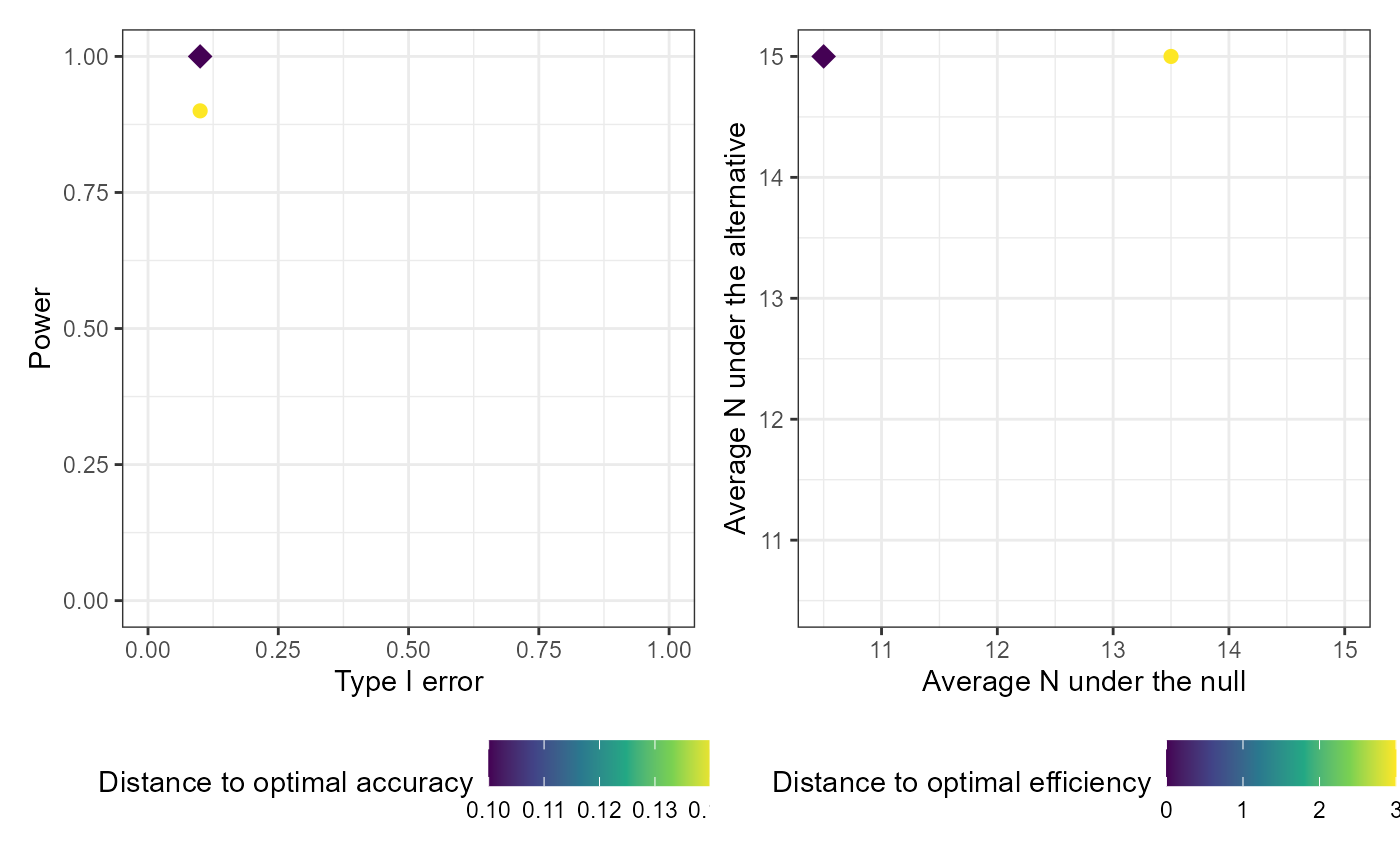Returns two interactive plotly plots (if plotly=TRUE) or two static ggplot2 plots (if plotly=FALSE) to compare results from various designs generated from a call to calibrate_thresholds based on various criteria, and to assist in selecting an optimal design.

## Usage

# S3 method for calibrate_thresholds
plot(x, type1_range = c(0, 1), minimum_power = 0, plotly = FALSE, ...)

## Arguments

x

an object of class 'calibrate_thresholds', usually returned by the calibrate_thresholds function

type1_range

a vector specifying the minimum and maximum acceptable type I error. Specify c(0, 1) to return the full range of resulting type I error. Defaults to c(0, 1)

minimum_power

a numeric between 0 and 1 specifying the minimum acceptable power. Specify 0 to return the full range of resulting power. Defaults to 0.

plotly

a logical indicator of whether you want the plots returned as interactive plotly plots or non-interactive ggplots. Defaults to FALSE.

...

unused

## Value

Plots of the average sample size under the null by the average sample size under the alternative, and the type I error by the power for designs meeting the specified type1_range and minimum_power

## Examples


# Setting S = 50 and nsim = 50 for speed
# In practice you would want a much larger sample and more simulations

set.seed(123)

# One-sample case
cal_tbl1 <- calibrate_thresholds(
p_null = 0.1,
p_alt = 0.4,
n = seq(5, 15, 5),
N = 15,
pp_threshold = c(0.85, 0.9),
ppp_threshold = c(0.1, 0.2),
S = 10,
nsim = 10
)
#> Joining, by = c("sim_num", "pp_threshold", "ppp_threshold")

plot(cal_tbl1, type1_range = c(0.01, 0.2), minimum_power = 0.7)